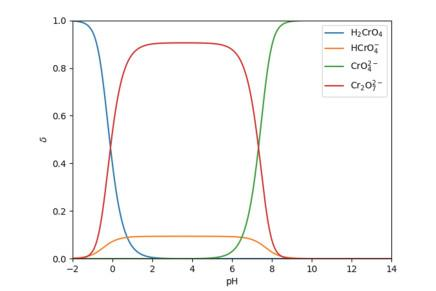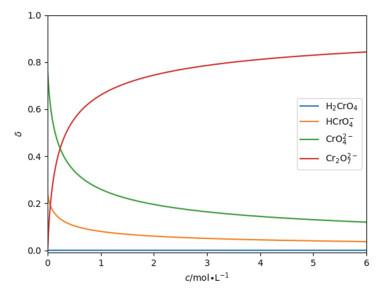## Application of Python in the Design of Analytical Chemistry Laboratory

Wang Hao2, Liu Hongyu,1, Wei Miaojin2

 基金资助: 2018年度中国科学技术大学本科教学研究项目.  2018xjyxm12Abstract

Taking the determination of the composition of calcium phosphate and the recovery of chromium waste liquor as the example, this paper discussed how to use Python to deal with the curve drawing and precise solution of the related problems in the design of analytical chemistry laboratory. Using Python for analysis, the process is more concise and intuitive, which can provide a better theoretical basis for experimental design.

Keywords： Python ; Analytical chemistry ; Experimental design

Wang Hao. Application of Python in the Design of Analytical Chemistry Laboratory. University Chemistry[J], 2020, 35(9): 198-204 doi:10.3866/PKU.DXHX201910037

## 1 基本处理方法

### 图1${\rm{Ca}}\;\;\;\;\;\;\;\;\;\;\;\;\;\;\;\;\;\;\;\;\;\;\;\;\left[ {{\rm{CaY}}} \right] + \left[ {{\rm{Ca}}} \right] = {c_{{\rm{Ca}}}} = 0.20/(V + {\rm{ }}80)$

${\rm{Bi}}\;\;\;\;\;\;\;\;\;\;\;\;\;\;\;\;\;\;\;\;\;\;\;\;\;\;\;\;\;\left[ {{\rm{BiY}}} \right] + \left[ {{\rm{Bi}}} \right] = {c_{{\rm{Bi}}}} = 0.38/(V + {\rm{ }}80)$

${\rm{EDTA}}\;\;\;\;\;\;\;\;\;\;\;\;\;\;\;\;\;\;\;\;\left[ {{\rm{CaY}}} \right] + \left[ {{\rm{BiY}}} \right] + [{\rm{Y''}}] = {c_{{\rm{EDTA}}}} = 0.02V/(V + 80)$

$[{\rm{Y}}'']$ = [Y]αY(H)${K_1}''$ = KCaY/αY(H)${K_2}''$ = KBiY/αY(H)

$由可得：[{\rm{Ca}}] = \frac{1}{{1 + [{\rm{Y}}'']{K_1}''}}\frac{{0.2}}{{V + 80}}$

$由可得：[{\rm{Bi}}] = \frac{1}{{1 + [{\rm{Y}}'']{K_2}''}}\frac{{0.38}}{{V + 80}}$

$解出V：V = \left( {\frac{{0.2[{\rm{Y}}'']{K_1}''}}{{1 + [{\rm{Y}}'']{K_1}''}} + \frac{{0.38[{\rm{Y}}'']{K_2}''}}{{1 + [{\rm{Y}}'']{K_2}''}} + 80[{\rm{Y}}'']} \right)\frac{1}{{0.02 - [{\rm{Y}}'']}}$

1. import matplotlib.pyplot as plt

2. import numpy as np

3. K1 = 4.9*10**10/(10**13.51)     #K1''

4. K2 = 8.71*10**27/(10**13.51)     #K2''

5. Vmin = 0     #加入的EDTA体积范围

6. Vmax = 40

7. py = np.linspace(-20, -2, 100000)      # py = -lg(y)，y or y_为[Y'']，作为自变量

8. y_ = []

9. V = []

10. pBi = []

11.

12. for i in py:      #计算V, pBi

13.   y_.append(10**i)

14.   y = y_[-1]

15.   V.append((0.2*K1*y/(1+K1*y)+0.38*K2*y/(1+K2*y)+80*y)/(0.02-y))  #即(6)式

16.    pBi.append(-np.log10(0.38/(V[-1]+80)/(1+K2*y)))     # pBi = -lg(Bi3+)，即(5)式

17.

18. def pfind(x, x0):     #查找求值

19.  for i in range(0, len(x)):

20.    if x[i] > = x0:

21.      return i

22.  print("No result!")

23.

24. print("pBi: ", pBi[pfind(V, 19.00)])      #理论终点

25. print("Et: ", (V[pfind(pBi, 5.40)]/19-1)*100)     #以二甲酚橙为指示剂的理论终点误差

26. print("pBiJump: ", pBi[pfind(V, 18.98)], pBi[pfind(V, 19.02)])

27.          #滴定突跃:19.00mL(1±0.1%)对应的pBi

28.

29. plt.plot(V, pBi, 'black')     #以V为横坐标，pBi为纵坐标，绘制pBi-V关系曲线

30. plt.xlim(Vmin, Vmax)

31. plt.xlabel("＄V＄/mL")

32. plt.ylabel(“＄pBi＄”)

33. plt.show()

$Et = \frac{{{V_{{\rm{ep}}}} - {V_{{\rm{sp}}}}}}{{{V_{{\rm{sp}}}}}} \times 100\% = - 0.1\%$，滴定曲线如图2所示。

### 图2## 2 与pH相关的物质形态问题

Cr(Ⅵ)在不同pH下的存在形式不同，氧化还原电对不同，主要存在以下平衡：

H2CrO4的电离：Ka1 = 0.18，Ka2 = 3.2 × 10−7

$2\mathrm{C}\mathrm{r}{\mathrm{O}}_{4}^{2-}+2{\mathrm{H}}^{+}\rightleftharpoons {\mathrm{C}\mathrm{r}}_{2}{\mathrm{O}}_{7}^{2-}+{\mathrm{H}}_{2}\mathrm{O} \ \ \ \ \ {K}_{3} = {10}^{14.69}$

$体系\text{MBE}： {c}_{\mathrm{C}\mathrm{r}}=\left[{\mathrm{H}}_{2}\mathrm{C}\mathrm{r}{\mathrm{O}}_{4}\right]+\left[\mathrm{H}\mathrm{C}\mathrm{r}{\mathrm{O}}_{4}^{-}\right]+\left[\mathrm{C}\mathrm{r}{\mathrm{O}}_{4}^{2-}\right]+2\left[{\mathrm{C}\mathrm{r}}_{2}{\mathrm{O}}_{7}^{2-}\right]$

Cr(Ⅵ)各形态浓度：

${K_{{\rm{a2}}}} = \frac{{[{\rm{CrO}}_4^{2 - }][{{\rm{H}}^{\rm{ + }}}]}}{{{\rm{[HCrO}}_4^ - ]}}，记{K_1} = \frac{1}{{{K_{{\rm{a2}}}}}} = {10^{6.49}} \Rightarrow {\rm{[HCrO}}_4^ - ] = {K_1}[{\rm{CrO}}_4^{2 - }][{{\rm{H}}^{\rm{ + }}}]$

${K_{{\rm{a1}}}}{K_{{\rm{a2}}}} = \frac{{[{\rm{CrO}}_4^{2 - }]{{[{{\rm{H}}^{\rm{ + }}}]}^2}}}{{{\rm{[}}{{\rm{H}}_2}{\rm{CrO}}_4^{}]}}，记{K_1} = \frac{1}{{{K_{{\rm{a1}}}}{K_{{\rm{a2}}}}}} = {10^{7.24}} \Rightarrow {\rm{[}}{{\rm{H}}_2}{\rm{CrO}}_4^{}] = {K_2}[{\rm{CrO}}_4^{2 - }]{[{{\rm{H}}^{\rm{ + }}}]^2}$

$\left[{\mathrm{C}\mathrm{r}}_{2}{\mathrm{O}}_{7}^{2-}\right]={K}_{3}{\left[\mathrm{C}\mathrm{r}{\mathrm{O}}_{4}^{2-}\right]}^{2}{\left[{\mathrm{H}}^{+}\right]}^{2}$

${c}_{\mathrm{C}\mathrm{r}}={K}_{2}\left[\mathrm{C}\mathrm{r}{\mathrm{O}}_{4}^{2-}\right]{\left[{\mathrm{H}}^{+}\right]}^{2}+{K}_{1}\left[\mathrm{C}\mathrm{r}{\mathrm{O}}_{4}^{2-}\right]\left[{\mathrm{H}}^{+}\right]+\left[\mathrm{C}\mathrm{r}{\mathrm{O}}_{4}^{2-}\right]+2{K}_{3}{\left[\mathrm{C}\mathrm{r}{\mathrm{O}}_{4}^{2-}\right]}^{2}{\left[{\mathrm{H}}^{+}\right]}^{2} \\记a = 2{K}_{3}{\left[{\mathrm{H}}^{+}\right]}^{2} ，b = {K}_{2}{\left[{\mathrm{H}}^{+}\right]}^{2}+{K}_{1}\left[{\mathrm{H}}^{+}\right]+1 \\则，{c}_{\mathrm{C}\mathrm{r}}=a{\left[\mathrm{C}\mathrm{r}{\mathrm{O}}_{4}^{2-}\right]}^{2}+b\left[\mathrm{C}\mathrm{r}{\mathrm{O}}_{4}^{2-}\right]$

${\rm{CrO}}_4^{2 - }分布分数：δ({\rm{CrO}}_4^{2 - }) = \frac{{[{\rm{CrO}}_4^{2 - }]}}{{{c_{{\rm{Cr}}}}}} = \frac{1}{{a[{\rm{CrO}}_4^{2 - }] + b}}$

cCr = 1.000 mol·L−1，由式(12)可求得[$\mathrm{C}\mathrm{r}{\mathrm{O}}_{4}^{2-}$] (此时也等于其分布分数)与[${\mathrm{H}}^{+}$]关系，

$[{\rm{CrO}}_4^{2 - }] = \frac{{ - b + \sqrt {{b^2} + 4a} }}{{2a}}$

### 图3$[{\rm{CrO}}_4^{2 - }] = \frac{{ - b + \sqrt {{b^2} + 4ac} }}{{2a}} \Rightarrow \delta ({\rm{CrO}}_4^{2 - }) = \frac{{ - b + \sqrt {{b^2} + 4ac} }}{{2ac}}$

### 图4### 图5$2\mathrm{H}\mathrm{C}\mathrm{r}{\mathrm{O}}_{4}^{-}\rightleftharpoons \left(2{\mathrm{H}}^{+}+2\mathrm{C}\mathrm{r}{\mathrm{O}}_{4}^{2-}\right)\rightleftharpoons {\mathrm{C}\mathrm{r}}_{2}{\mathrm{O}}_{7}^{2-}+{\mathrm{H}}_{2}\mathrm{O}$

$K = \frac{{[{\rm{C}}{{\rm{r}}_2}{\rm{O}}_7^{2 - }]}}{{{{{\rm{[HCrO}}_4^ - ]}^2}}} = {K_{{\rm{a2}}}}{K_{\rm{3}}} = 50.15$

${\mathrm{C}\mathrm{r}}_{2}{\mathrm{O}}_{7}^{2-}、\mathrm{H}\mathrm{C}\mathrm{r}{\mathrm{O}}_{4}^{-}$间的平衡关系与pH无关，相互关联，所以有图34中的相似性，浓度较大时${\mathrm{C}\mathrm{r}}_{2}{\mathrm{O}}_{7}^{2-}$分布分数更大，浓度极小时关系反转，在图45中有所体现。

## 参考文献 原文顺序 文献年度倒序 文中引用次数倒序 被引期刊影响因子

/

 〈〉